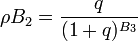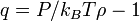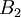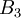# Pair stress approximation$\rho B_2 = \frac{q}{(1+q)^{B_3}}$
where$q= P/k_BT\rho -1$,$B_2$ is the second virial coefficient and$B_3$ is the third virial coefficient.